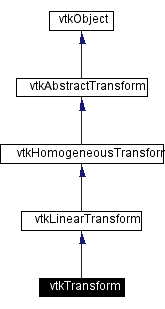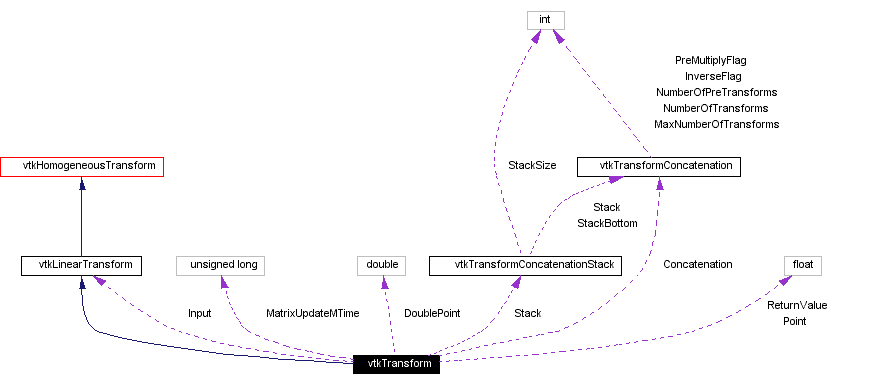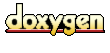Main Page   Class Hierarchy   Alphabetical List   Compound List   File List   Compound Members   File Members   Related Pages

# vtkTransform Class Reference

describes linear transformations via a 4x4 matrix. More...

`#include <vtkTransform.h>`

Inheritance diagram for vtkTransform:[legend]
Collaboration diagram for vtkTransform:[legend]
List of all members.

## Public Methods

virtual const char * GetClassName ()
virtual int IsA (const char *type)
void PrintSelf (ostream &os, vtkIndent indent)
void Identity ()
void Inverse ()
void Translate (double x, double y, double z)
void Translate (const double x)
void Translate (const float x)
void RotateWXYZ (double angle, double x, double y, double z)
void RotateWXYZ (double angle, const double axis)
void RotateWXYZ (double angle, const float axis)
void RotateX (double angle)
void RotateY (double angle)
void RotateZ (double angle)
void Scale (double x, double y, double z)
void Scale (const double s)
void Scale (const float s)
void SetMatrix (vtkMatrix4x4 *matrix)
void SetMatrix (const double elements)
void Concatenate (vtkMatrix4x4 *matrix)
void Concatenate (const double elements)
void Concatenate (vtkLinearTransform *transform)
void PreMultiply ()
void PostMultiply ()
void GetOrientation (double orient)
void GetOrientation (float orient)
float * GetOrientation ()
void GetOrientationWXYZ (double wxyz)
void GetOrientationWXYZ (float wxyz)
float * GetOrientationWXYZ ()
void GetPosition (double pos)
void GetPosition (float pos)
float * GetPosition ()
void GetScale (double scale)
void GetScale (float scale)
float * GetScale ()
void GetInverse (vtkMatrix4x4 *inverse)
void GetTranspose (vtkMatrix4x4 *transpose)
void Push ()
void Pop ()
void SetInput (vtkLinearTransform *input)
vtkLinearTransformGetInput ()
int CircuitCheck (vtkAbstractTransform *transform)
vtkAbstractTransformGetInverse ()
vtkAbstractTransformMakeTransform ()
unsigned long GetMTime ()
void MultiplyPoint (const float in, float out)
void MultiplyPoint (const double in, double out)
void MultiplyPoints (vtkPoints *inPts, vtkPoints *outPts)
void MultiplyVectors (vtkVectors *inVectors, vtkVectors *outVectors)
void MultiplyNormals (vtkNormals *inNormals, vtkNormals *outNormals)
void Transpose ()
virtual void SetPoint (float, float, float, float)
virtual void SetPoint (float)
virtual void SetDoublePoint (double, double, double, double)
virtual void SetDoublePoint (double)
float * GetPoint ()
double * GetDoublePoint ()
void GetPoint (float p)
void Multiply4x4 (vtkMatrix4x4 *a, vtkMatrix4x4 *b, vtkMatrix4x4 *c)
void Multiply4x4 (const double a, const double b, double c)
void Multiply4x4 (vtkMatrix4x4 &a, vtkMatrix4x4 &b, vtkMatrix4x4 &c)
void Concatenate (vtkMatrix4x4 &matrix)
void SetMatrix (vtkMatrix4x4 &m)
void GetTranspose (vtkMatrix4x4 &transpose)
void GetInverse (vtkMatrix4x4 &inverse)
void GetOrientation (float *prx, float *pry, float *prz)
void GetOrientation (float &rx, float &ry, float &rz)
void GetPosition (float *px, float *py, float *pz)
void GetPosition (float &x, float &y, float &z)
void GetScale (float *psx, float *psy, float *psz)
void GetScale (float &x, float &y, float &z)

## Static Public Methods

vtkTransform * New ()
int IsTypeOf (const char *type)
vtkTransform * SafeDownCast (vtkObject *o)

## Protected Methods

vtkTransform ()
~vtkTransform ()
vtkTransform (const vtkTransform &t)
void operator= (const vtkTransform &)
void InternalDeepCopy (vtkAbstractTransform *t)
void InternalUpdate ()

## Protected Attributes

vtkLinearTransformInput
vtkTransformConcatenationConcatenation
vtkTransformConcatenationStackStack
unsigned long MatrixUpdateMTime
float Point 
double DoublePoint 
float ReturnValue 

## Detailed Description

describes linear transformations via a 4x4 matrix.

Date:
2000/12/10 20:08:19
Revision:
1.73

A vtkTransform can be used to describe the full range of linear (also known as affine) coordinate transformations in three dimensions, which are internally represented as a 4x4 homogeneous transformation matrix. When you create a new vtkTransform, it is always initialized to the identity transformation.

The SetInput() method allows you to set another transform, instead of the identity transform, to be the base transformation. There is a pipeline mechanism to ensure that when the input is modified, the current transformation will be updated accordingly. This pipeline mechanism is also supported by the Concatenate() method.

Most of the methods for manipulating this transformation, e.g. Translate, Rotate, and Concatenate, can operate in either PreMultiply (the default) or PostMultiply mode. In PreMultiply mode, the translation, concatenation, etc. will occur before any transformations which are represented by the current matrix. In PostMultiply mode, the additional transformation will occur after any transformations represented by the current matrix.

This class performs all of its operations in a right handed coordinate system with right handed rotations. Some other graphics libraries use left handed coordinate systems and rotations.

vtkPerspectiveTransform vtkGeneralTransform vtkMatrix4x4 vtkTransformCollection vtkTransformFilter vtkTransformPolyDataFilter vtkImageReslice
Examples:
vtkTransform (examples)

Definition at line 81 of file vtkTransform.h.

## Constructor & Destructor Documentation

 vtkTransform::vtkTransform ( ) ` [protected]`

 vtkTransform::~vtkTransform ( ) ` [protected]`

 vtkTransform::vtkTransform ( const vtkTransform & t ) ` [protected]`

## Member Function Documentation

 vtkTransform* vtkTransform::New ( ) ` [static]`
 Create an object with Debug turned off, modified time initialized to zero, and reference counting on. Reimplemented from vtkObject.

 virtual const char* vtkTransform::GetClassName ( ) ` [virtual]`
 Return the class name as a string. This method is defined in all subclasses of vtkObject with the vtkTypeMacro found in vtkSetGet.h. Reimplemented from vtkLinearTransform.

 int vtkTransform::IsTypeOf ( const char * type ) ` [static]`
 Return 1 if this class type is the same type of (or a subclass of) the named class. Returns 0 otherwise. This method works in combination with vtkTypeMacro found in vtkSetGet.h. Reimplemented from vtkLinearTransform.

 virtual int vtkTransform::IsA ( const char * type ) ` [virtual]`
 Return 1 if this class is the same type of (or a subclass of) the named class. Returns 0 otherwise. This method works in combination with vtkTypeMacro found in vtkSetGet.h. Reimplemented from vtkLinearTransform.

 vtkTransform* vtkTransform::SafeDownCast ( vtkObject * o ) ` [static]`
 Will cast the supplied object to vtkObject* is this is a safe operation (i.e., a safe downcast); otherwise NULL is returned. This method is defined in all subclasses of vtkObject with the vtkTypeMacro found in vtkSetGet.h. Reimplemented from vtkLinearTransform. Referenced by vtkTransformCollection::GetNextItem().

 void vtkTransform::PrintSelf ( ostream & os, vtkIndent indent ) ` [virtual]`
 Methods invoked by print to print information about the object including superclasses. Typically not called by the user (use Print() instead) but used in the hierarchical print process to combine the output of several classes. Reimplemented from vtkLinearTransform.

 void vtkTransform::Identity ( )
 Set the transformation to the identity transformation. If the transform has an Input, then the transformation will be reset so that it is the same as the Input. Reimplemented from vtkAbstractTransform.

 void vtkTransform::Inverse ( ) ` [virtual]`
 Invert the transformation. This will also set a flag so that the transformation will use the inverse of its Input, if an Input has been set. Reimplemented from vtkAbstractTransform.

 void vtkTransform::Translate ( double x, double y, double z ) ` [inline]`
 Create a translation matrix and concatenate it with the current transformation according to PreMultiply or PostMultiply semantics. Definition at line 100 of file vtkTransform.h.

 void vtkTransform::Translate ( const double x ) ` [inline]`
 Definition at line 102 of file vtkTransform.h.

 void vtkTransform::Translate ( const float x ) ` [inline]`
 Definition at line 103 of file vtkTransform.h.

 void vtkTransform::RotateWXYZ ( double angle, double x, double y, double z ) ` [inline]`
 Create a rotation matrix and concatenate it with the current transformation according to PreMultiply or PostMultiply semantics. The angle is in degrees, and (x,y,z) specifies the axis that the rotation will be performed around. Definition at line 109 of file vtkTransform.h.

 void vtkTransform::RotateWXYZ ( double angle, const double axis ) ` [inline]`
 Definition at line 111 of file vtkTransform.h.

 void vtkTransform::RotateWXYZ ( double angle, const float axis ) ` [inline]`
 Definition at line 113 of file vtkTransform.h.

 void vtkTransform::RotateX ( double angle ) ` [inline]`
 Create a rotation matrix about the X, Y, or Z axis and concatenate it with the current transformation according to PreMultiply or PostMultiply semantics. The angle is expressed in degrees. Definition at line 119 of file vtkTransform.h.

 void vtkTransform::RotateY ( double angle ) ` [inline]`
 Definition at line 120 of file vtkTransform.h.

 void vtkTransform::RotateZ ( double angle ) ` [inline]`
 Definition at line 121 of file vtkTransform.h.

 void vtkTransform::Scale ( double x, double y, double z ) ` [inline]`
 Create a scale matrix (i.e. set the diagonal elements to x, y, z) and concatenate it with the current transformation according to PreMultiply or PostMultiply semantics. Definition at line 126 of file vtkTransform.h.

 void vtkTransform::Scale ( const double s ) ` [inline]`
 Definition at line 128 of file vtkTransform.h.

 void vtkTransform::Scale ( const float s ) ` [inline]`
 Definition at line 129 of file vtkTransform.h.

 void vtkTransform::SetMatrix ( vtkMatrix4x4 * matrix ) ` [inline]`
 Set the current matrix directly. This actually calls Identity(), followed by Concatenate(matrix). Definition at line 133 of file vtkTransform.h.

 void vtkTransform::SetMatrix ( const double elements ) ` [inline]`
 Definition at line 135 of file vtkTransform.h.

 void vtkTransform::Concatenate ( vtkMatrix4x4 * matrix ) ` [inline]`
 Concatenates the matrix with the current transformation according to PreMultiply or PostMultiply semantics. Definition at line 140 of file vtkTransform.h.

 void vtkTransform::Concatenate ( const double elements ) ` [inline]`
 Definition at line 142 of file vtkTransform.h.

 void vtkTransform::Concatenate ( vtkLinearTransform * transform )
 Concatenate the specified transform with the current transformation according to PreMultiply or PostMultiply semantics. The concatenation is pipelined, meaning that if any of the transformations are changed, even after Concatenate() is called, those changes will be reflected when you call TransformPoint().

 void vtkTransform::PreMultiply ( ) ` [inline]`
 Sets the internal state of the transform to PreMultiply. All subsequent operations will occur before those already represented in the current transformation. In homogeneous matrix notation, M = M*A where M is the current transformation matrix and A is the applied matrix. The default is PreMultiply. Definition at line 157 of file vtkTransform.h.

 void vtkTransform::PostMultiply ( ) ` [inline]`
 Sets the internal state of the transform to PostMultiply. All subsequent operations will occur after those already represented in the current transformation. In homogeneous matrix notation, M = A*M where M is the current transformation matrix and A is the applied matrix. The default is PreMultiply. Definition at line 166 of file vtkTransform.h.

 void vtkTransform::GetOrientation ( double orient )
 Get the x, y, z orientation angles from the transformation matrix as an array of three floating point values.

 void vtkTransform::GetOrientation ( float orient ) ` [inline]`
 Definition at line 173 of file vtkTransform.h.

 float* vtkTransform::GetOrientation ( ) ` [inline]`
 Definition at line 176 of file vtkTransform.h.

 void vtkTransform::GetOrientationWXYZ ( double wxyz )
 Return the wxyz angle+axis representing the current orientation.

 void vtkTransform::GetOrientationWXYZ ( float wxyz ) ` [inline]`
 Definition at line 181 of file vtkTransform.h.

 float* vtkTransform::GetOrientationWXYZ ( ) ` [inline]`
 Definition at line 184 of file vtkTransform.h.

 void vtkTransform::GetPosition ( double pos )
 Return the position from the current transformation matrix as an array of three floating point numbers. This is simply returning the translation component of the 4x4 matrix.

 void vtkTransform::GetPosition ( float pos ) ` [inline]`
 Definition at line 191 of file vtkTransform.h.

 float* vtkTransform::GetPosition ( ) ` [inline]`
 Definition at line 194 of file vtkTransform.h.

 void vtkTransform::GetScale ( double scale )
 Return the scale factors of the current transformation matrix as an array of three float numbers. These scale factors are not necessarily about the x, y, and z axes unless unless the scale transformation was applied before any rotations.

 void vtkTransform::GetScale ( float scale ) ` [inline]`
 Definition at line 202 of file vtkTransform.h.

 float* vtkTransform::GetScale ( ) ` [inline]`
 Definition at line 205 of file vtkTransform.h.

 void vtkTransform::GetInverse ( vtkMatrix4x4 * inverse )
 Return a matrix which is the inverse of the current transformation matrix.

 void vtkTransform::GetTranspose ( vtkMatrix4x4 * transpose )
 Return a matrix which is the transpose of the current transformation matrix. This is equivalent to the inverse if and only if the transformation is a pure rotation with no translation or scale.

 void vtkTransform::Push ( ) ` [inline]`
 Pushes the current transformation onto the transformation stack. Definition at line 218 of file vtkTransform.h.

 void vtkTransform::Pop ( ) ` [inline]`
 Deletes the transformation on the top of the stack and sets the top to the next transformation on the stack. Definition at line 225 of file vtkTransform.h.

 void vtkTransform::SetInput ( vtkLinearTransform * input )
 Set the input for this transformation. This will be used as the base transformation if it is set. This method allows you to build a transform pipeline: if the input is modified, then this transformation will automatically update accordingly.

 vtkLinearTransform* vtkTransform::GetInput ( ) ` [inline]`
 Definition at line 234 of file vtkTransform.h.

 int vtkTransform::CircuitCheck ( vtkAbstractTransform * transform ) ` [virtual]`
 Check for self-reference. Will return true if concatenating with the specified transform, setting it to be our inverse, or setting it to be our input will create a circular reference. CircuitCheck is automatically called by SetInput(), SetInverse(), and Concatenate(vtkXTransform *). Avoid using this function, it is experimental. Reimplemented from vtkAbstractTransform.

 vtkAbstractTransform* vtkTransform::GetInverse ( ) ` [inline]`
 Get the inverse of this transform. If you modify this transform, the returned inverse transform will automatically update. If you want the inverse of a vtkTransform, you might want to use GetLinearInverse() instead which will type cast the result from vtkAbstractTransform to vtkLinearTransform. Reimplemented from vtkAbstractTransform. Definition at line 246 of file vtkTransform.h.

 vtkAbstractTransform* vtkTransform::MakeTransform ( ) ` [virtual]`
 Make a new transform of the same type. Reimplemented from vtkAbstractTransform.

 unsigned long vtkTransform::GetMTime ( ) ` [virtual]`
 Override GetMTime to account for input and concatenation. Reimplemented from vtkAbstractTransform.

 void vtkTransform::MultiplyPoint ( const float in, float out ) ` [inline]`
 Use this method only if you wish to compute the transformation in homogeneous (x,y,z,w) coordinates, otherwise use TransformPoint(). This method calls this->GetMatrix()->MultiplyPoint(). Definition at line 258 of file vtkTransform.h.

 void vtkTransform::MultiplyPoint ( const double in, double out ) ` [inline]`
 Definition at line 260 of file vtkTransform.h.

 void vtkTransform::MultiplyPoints ( vtkPoints * inPts, vtkPoints * outPts ) ` [inline]`
 These methods are obsolete. Use TransformPoints, TransformVectors, or TransformNormals instead. Definition at line 266 of file vtkTransform.h.

 void vtkTransform::MultiplyVectors ( vtkVectors * inVectors, vtkVectors * outVectors ) ` [inline]`
 Definition at line 268 of file vtkTransform.h.

 void vtkTransform::MultiplyNormals ( vtkNormals * inNormals, vtkNormals * outNormals ) ` [inline]`
 Definition at line 270 of file vtkTransform.h.

 void vtkTransform::Transpose ( ) ` [inline]`
 This method is deprecated because 1) it can turn a linear transformation matrix into a perspective transform matrix and 2) there is no way for the transformation pipeline to properly handle it. If the transformation is a pure rotation, then use GetInverse(), otherwise try to work around this method by using GetTranspose(). Definition at line 279 of file vtkTransform.h.

 virtual void vtkTransform::SetPoint ( float , float , float , float ) ` [virtual]`
 Do not use these functions -- they are here only to provide compatibility with legacy code. Use TransformPoint() or GetMatrix()->MultiplyPoint() instead. Returns the result of multiplying the currently set Point by the current transformation matrix. Point is expressed in homogeneous coordinates. The setting of the PreMultiplyFlag will determine if the Point is Pre or Post multiplied.

 virtual void vtkTransform::SetPoint ( float  ) ` [virtual]`

 virtual void vtkTransform::SetDoublePoint ( double , double , double , double ) ` [virtual]`

 virtual void vtkTransform::SetDoublePoint ( double  ) ` [virtual]`

 float* vtkTransform::GetPoint ( )

 double* vtkTransform::GetDoublePoint ( )

 void vtkTransform::GetPoint ( float p )

 void vtkTransform::Multiply4x4 ( vtkMatrix4x4 * a, vtkMatrix4x4 * b, vtkMatrix4x4 * c ) ` [inline]`
 For legacy compatibility. Do not use. Definition at line 302 of file vtkTransform.h.

 void vtkTransform::Multiply4x4 ( const double a, const double b, double c ) ` [inline]`
 Definition at line 304 of file vtkTransform.h.

 void vtkTransform::Multiply4x4 ( vtkMatrix4x4 & a, vtkMatrix4x4 & b, vtkMatrix4x4 & c ) ` [inline]`
 Definition at line 306 of file vtkTransform.h.

 void vtkTransform::Concatenate ( vtkMatrix4x4 & matrix ) ` [inline]`
 Definition at line 308 of file vtkTransform.h.

 void vtkTransform::SetMatrix ( vtkMatrix4x4 & m ) ` [inline]`
 Definition at line 310 of file vtkTransform.h.

 void vtkTransform::GetTranspose ( vtkMatrix4x4 & transpose ) ` [inline]`
 Definition at line 312 of file vtkTransform.h.

 void vtkTransform::GetInverse ( vtkMatrix4x4 & inverse ) ` [inline]`
 Definition at line 314 of file vtkTransform.h.

 void vtkTransform::GetOrientation ( float * prx, float * pry, float * prz ) ` [inline]`
 Definition at line 317 of file vtkTransform.h.

 void vtkTransform::GetOrientation ( float & rx, float & ry, float & rz ) ` [inline]`
 Definition at line 321 of file vtkTransform.h.

 void vtkTransform::GetPosition ( float * px, float * py, float * pz ) ` [inline]`
 Definition at line 323 of file vtkTransform.h.

 void vtkTransform::GetPosition ( float & x, float & y, float & z ) ` [inline]`
 Definition at line 327 of file vtkTransform.h.

 void vtkTransform::GetScale ( float * psx, float * psy, float * psz ) ` [inline]`
 Definition at line 329 of file vtkTransform.h.

 void vtkTransform::GetScale ( float & x, float & y, float & z ) ` [inline]`
 Definition at line 333 of file vtkTransform.h.

 void vtkTransform::operator= ( const vtkTransform & ) ` [inline, protected]`
 Definition at line 341 of file vtkTransform.h.

 void vtkTransform::InternalDeepCopy ( vtkAbstractTransform * t ) ` [protected, virtual]`
 Perform any subclass-specific DeepCopy. Reimplemented from vtkHomogeneousTransform.

 void vtkTransform::InternalUpdate ( ) ` [protected, virtual]`
 Perform any subclass-specific Update. Reimplemented from vtkAbstractTransform.

## Member Data Documentation

 vtkLinearTransform* vtkTransform::Input` [protected]`
 Definition at line 347 of file vtkTransform.h.

 vtkTransformConcatenation* vtkTransform::Concatenation` [protected]`
 Definition at line 348 of file vtkTransform.h.

 vtkTransformConcatenationStack* vtkTransform::Stack` [protected]`
 Definition at line 349 of file vtkTransform.h.

 unsigned long vtkTransform::MatrixUpdateMTime` [protected]`
 Definition at line 355 of file vtkTransform.h.

 float vtkTransform::Point` [protected]`
 Definition at line 360 of file vtkTransform.h.

 double vtkTransform::DoublePoint` [protected]`
 Definition at line 361 of file vtkTransform.h.

 float vtkTransform::ReturnValue` [protected]`
 Definition at line 362 of file vtkTransform.h.

The documentation for this class was generated from the following file:

Generated on Wed Nov 21 13:01:05 2001 for VTK by1.2.11.1 written by Dimitri van Heesch, © 1997-2001